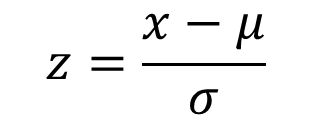# Study Guide for Problem # 3

Tanzima Mursalin

I will be explaining problem # 3

3) In a recent contest, the mean score was 210, and the standard deviation was 25.

a) Find the z score of John who scored 190

b) Find the z score of Bill who scored 270.

c) If Mary had a z score of 1.25, what was Mary’s score?

Step 1: First list all the information thats given: Mean or Mu= 210 & Standard Deviation or theta= 25

Solving part (a)

To find the z score we have to use a formula which isX = 190, which is John’s score.

Next step is to plug in all the values and solve for z.

Z = 190 – 210/ 25    Ans: – 0.8

Solving part (b)

For part b, we have to follow the same exact steps as part a.

X = 270, which is Bill’s score.

Z = 270 – 210/ 25    Ans: 2.4

Solving part (c)

In part (c) the z score is given and we have to find the score. to find the score the formula we have to use is X = µ + zӨ

Mary’s z score is 1.25 and to find her score you have to solve for x.

x = 210 + (1.25)(25)    Ans: 241.25